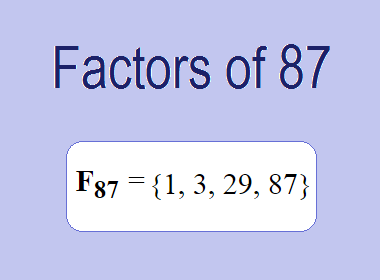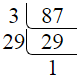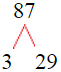# Factors of 87The factors of 87 are 1, 3, 29, and 87 i.e. F87 = {1, 3, 29, 87}. The factors of 87 are all the numbers that can divide 87 without leaving a remainder.

We can check if these numbers are factors of 87 by dividing 87 by each of them. If the result is a whole number, then the number is a factor of 87. Let's do this for each of the numbers listed above:

·        1 is a factor of 87 because 87 divided by 1 is 87.

·        3 is a factor of 87 because 87 divided by 3 is 29.

·        29 is a factor of 87 because 87 divided by 29 is 3.

·        87 is a factor of 87 because 87 divided by 87 is 1.

## How to Find Factors of 87?

1 and the number itself are the factors of every number. So, 1 and 87 are two factors of 87. To find the other factors of 87, we can start by dividing 87 by the numbers between 1 and 87. If we divide 87 by 2, we get a remainder of 1. Therefore, 2 is not a factor of 87. If we divide 87 by 3, we get a remainder of 0. Therefore, 3 is a factor of 87.

Next, we can check if 4 is a factor of 87. If we divide 87 by 4, we get a remainder of 3. Therefore, 4 is not a factor of 87. We can continue this process for all the possible factors of 87.

Through this process, we can find that the factors of 87 are 1, 3, 29, and 87. These are the only numbers that can divide 87 without leaving a remainder.

********************

********************

## Properties of the Factors of 87

The factors of 87 have some interesting properties. One of the properties is that the sum of the factors of 87 is equal to 120. We can see this by adding all the factors of 87 together:

1 + 3 + 29 + 87 = 120

Another property of the factors of 87 is that they are all odd numbers. This is because 87 is an odd number, and any even number cannot divide an odd number without leaving a remainder.

Another property of the factors of 87 is that the prime factors of 87 are 3 and 29 only.

## Applications of the Factors of 87

The factors of 87 have several applications in mathematics. One of the applications is in finding the highest common factor (HCF) of two or more numbers. The HCF is the largest factor that two or more numbers have in common. For example, to find the HCF of 87 and 29, we need to find the factors of both numbers and identify the largest factor they have in common. The factors of 87 are 1, 3, 29, and 87. The factors of 29 are 1 and 29. The largest factor that they have in common is 29. Therefore, the HCF of 87 and 29 is 29.

Another application of the factors of 87 is in prime factorization. Prime factorization is the process of expressing a number as the product of its prime factors. The prime factors of 87 are 3 and 29. We can express 87 as:

87 = 3 × 29

We can do prime factorization by division and factor tree method also. Here is the prime factorization of 87 by division method,87 = 3 × 29

Here is the prime factorization of 87 by the factor tree method,87 = 3 × 29

## Conclusion

The factors of 87 are the numbers that can divide 87 without leaving a remainder. The factors of 87 are 1, 3, 29, and 87. The factors of 87 have some interesting properties, such as being odd numbers and having a sum of 120. The factors of 87 have several applications in mathematics, such as finding the highest common factor and prime factorization.